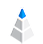September 15, 20204 min read

# Confusion Matrix Explained

The confusion matrix is a table that helps to visualize and understand the performance of supervised algorithms where the target is to determine a logical value. In this article, we will demonstrate the confusion matrix on a prediction algorithm of a decision tree. It shows how effective the predictive algorithm is and how many times it hits the correct or wrong outcome.

The name might be scary as it can be confusing for the first sight, but it is more related to the cells’ values.

## What is the Confusion Matrix?

The confusion matrix stands with a decision tree (prediction tree in AnswerMiner). Because of this reason, in the following guide, we will write about the tree as well. If the tree is Batman, the matrix is Robin, or if the tree is Captain America, the matrix is Bucky Barnes (just to favor for both DC and Marvel fans).

Back to statistics, the average should always be displayed with a standard deviation to cover reality. This is the same with the tree where the matrix should be displayed with it, or at least the information should be included in the interpretation. Le’ts get back to this later. There are some “definitions” that you need to know before you dig deeper.

## How to read the Confusion Matrix?

### Training and testing

When using a prediction model, there will be two groups of data. One that the algorithm analyzes and learns the patterns on and the other where it uses what it learned on the first one. In AnswerMiner, the prediction tree can train on 70% of the data and test efficiency on 30%. Because of that, you will see the test result in the confusion matrix about the 30% data group.

### Actual and Predicted class

The followings are the most important definitions related to the confusion matrix. Comparing the predicted and the actual values will give the efficiency of the algorithm.

• The Actual class: The actual class includes the true/real/actual values that we used as a reference when we evaluate the prediction.

• The Predicted class: The predicted class includes the values that we predicted with the algorithm.

### The values in the cells

As the name itself describes, it is a matrix that includes four cells. Each cell has a number, which represents the number of cases. Those cases can be True-positive, True-negative, False-positive, and False-negative. To understand the meaning of these values, let’s see the following example.

### Example

We want to predict if the given person is pregnant or not. In this case, the target value will be pregnant=yes in the prediction tree. The matrix will look like this.• True-negative: The predicted=negative and actual=negative, so it’s true what you have predicted.You predicted that a man is not pregnant, and he actually is not.

• False-negative: The predicted=negative and actual=positive, so it’s false what you have predicted.You predicted that a woman is not pregnant, but she actually is.

• False-positive: The predicted=positive and actual=negative, so it’s false what you have predicted.You predicted that a man is pregnant, but he actually is not.

• True-positive: The positive and actual=positive, so it’s true what you have predicted.You predicted that a woman is pregnant, and she actually is.## Specificity, Sensitivity, and Accuracy

Based on the predicted and actual results, there are some measurement numbers you will be able to describe the outcome of the algorithm.

The Specificity, also known as Selectivity or True Negative Rate (TNR)The Sensitivity, also known as Recall, Hit rate, or True Positive Rate (TPR).The Negative Predicted ValueThe Positive Predicted ValueTotal Accuracy, also known as ACC, which is the ratio of the correctly predicted cases out of all tested cases.## Cut-off value

The prediction algorythm estimates the probability (in percentage 0-100) of describing a group true or false. From that estimation, the algorythm creates a concrete yes or no value.

By default, above 50% results “yes”, below 50% results “no”. Changing this cutoff treshold value, we can make the prediction more optimistic or pessimistic. So that, if the cutoff value is 90%, we only consider a group of data to be true, if our algorythm gives at least 90% chanche that the elements of the group are true.

Cookies help us in delivering our service. You consent to our cookies and you agree to our privacy policy and cookie policy if you continue to use our website. Learn more about our privacy policy here and cookie policy here.